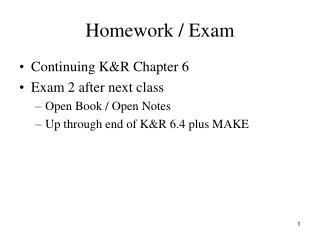Download PresentationHomework / Exam

# Homework / Exam - PowerPoint PPT PresentationDownload Presentation## Homework / Exam

- - - - - - - - - - - - - - - - - - - - - - - - - - - E N D - - - - - - - - - - - - - - - - - - - - - - - - - - -
##### Presentation Transcript

1. Homework / Exam • Continuing K&R Chapter 6 • Exam 2 after next class • Open Book / Open Notes • Up through end of K&R 6.4 plus MAKE

2. Review structs struct point { int x; int y; } pt; struct point pt1, pt2; struct point maxpt = {320, 200};

3. Review structs struct rect { struct point pt1; struct point pt2; };  Note: must have ; following } struct rect box;

4. Review structs box.pt1.x = 5; box.pt1.y = 10; box.pt2.x = 10; box.pt2.y = 20; area = (box.pt2.x – box.pt1.x) * (box.pt2.y – box.pt1.y);

5. What can we do with a struct? • Reference members box.pt2.x = box.pt1.x + width; • Assign as a unit pt2 = pt1; • Create a pointer to it struct point *ppt1; ppt1 = &pt1;

6. What can we do with a struct? • Not legal to compare structs if (pt1 == pt2) …  INVALID • Must be done as: if (pt1.x == pt2.x && pt1.y == pt2.y) …

7. structs and Functions, K&R 6.2 /* ptinrect: if point p in rect r, return 1 else return 0 note: slightly different from K&R example */ int ptinrect (struct point p, struct rect r) { return p.x >= r.pt1.x && p.x <= r.pt2.x && p.y >= r.pt1.y && p.y <= r.pt2.y; }

8. Arrays of structs, K&R 6.3 • Multiple related arrays char * keyword[NKEYS]; int keycount[NKEYS]; • Can be implemented as an array of structs struct key { char *word; int count; } keytab [NKEYS];

9. Arrays of structs • Alternative array of structs implementation struct key { char *word; int count; }; struct key keytab[NKEYS];

10. Arrays of structs • Initialization for an array of structs struct key { char *word; int count; } keytab[ ] = { “auto”, 0, … “while”, 0 }; /* NKEYS is dynamically derived */

11. Size of structs • sizeof is a compile-time unary operator • Can be used to get integer (actually size_t): sizeof object sizeof (type name) • Applied to structs #define NKEYS (sizeof keytab / sizeof (struct key)) #define NKEYS (sizeof keytab / sizeof keytab)

12. Pointers to structs, K&R 6.4 • Declare and initialize a pointer to struct struct point p; struct point *pp = &p; • Refer to members of struct p via pointer pp (*pp).x and (*pp).y  See precedence • More commonly done as: pp->x and pp->y  See precedence

13. Pointers to structs struct string { int len; char *cp; } *p; Expression Same as Value / Effect ++p->len ++(p->len) increments len *p->cp *(p->cp) value is a char *p->cp++ *((p->cp)++) value is a char increments cp

14. Pointers to structs /* ptinrect: (pointer version) if point p in rect r, return 1 else return 0 */ int ptinrect (struct point *pp, struct rect *rp) { return pp->x >= rp->pt1.x && pp->x <= rp->pt2.x && pp->y >= rp->pt1.y && pp->y <= rp->pt2.y; }